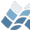NV5 GEOSPATIAL SOFTWAREMATCH_ND

MATCH_ND

## Purpose

For each arbitrarily-dimensioned point in one vector, determines the closest
point in a second vector. Method is to take the list returned by MATCHALL_ND
and narrow it down to the closest match.

Astro

## Calling Sequence

Result = MATCH_ND(P1, P2, MaxDistance)

## Inputs

P1: N1 x D array of D-dimensional coordinates.
P2: N2 x D array of D-dimensional coordinates.
MaxDistance: Maximum D-dimensional distance.

## Keyword Parameters

MINDIST: Optional output containing an array of the actual distance
to the closest match for each element of P1.
ONE_TO_ONE: Enforces one-to-one matching. By default, matching can
be many-to-one, i.e. one entry in P2 can be the
closest match to several entries in P1. If
/ONE_TO_ONE is given, then each multiple entry in P2
is first assigned to its closest point in P1. Then any
entries in P1 that have lost their match are assigned
the next closest point within MaxDistance. This process is
iterated until all points in P1 are matched to a
unique point in P2 or there are no more points
within MaxDistance.

## Outputs

The function returns an array with one entry for each P1
element containing the index in P2 that is closest, or -1 if
there are none within MaxDistance.

## Example

na = 10
nb = 1000
a = randomn(seed, na, 3)
b = 2. * randomu(seed, nb, 3) - 1
matches = match_nd(a, b, 0.3)
iplot, /scatter, /iso, b[*,0], b[*,1], b[*,2]
iplot, /overplot, /scatter, a[*,0], a[*,1], a[*,2], sym_index=6, \$
sym_color=[255,0,0]
for i=0L, na-1 do if matches[i] ne -1 then \$
iplot, /overplot, [a[i,0], b[matches[i],0]], [a[i,1], b[matches[i],1]], \$
[a[i,2], b[matches[i],2]], sym_index=0, color=[255,0,0]

## Modification History

Written by: Jeremy Bailin
10 June 2008 Public release in JBIU as WITHINSPHRAD
24 April 2009 Vectorized as WITHINSPHRAD_VEC
25 April 2009 Polished to improve memory use